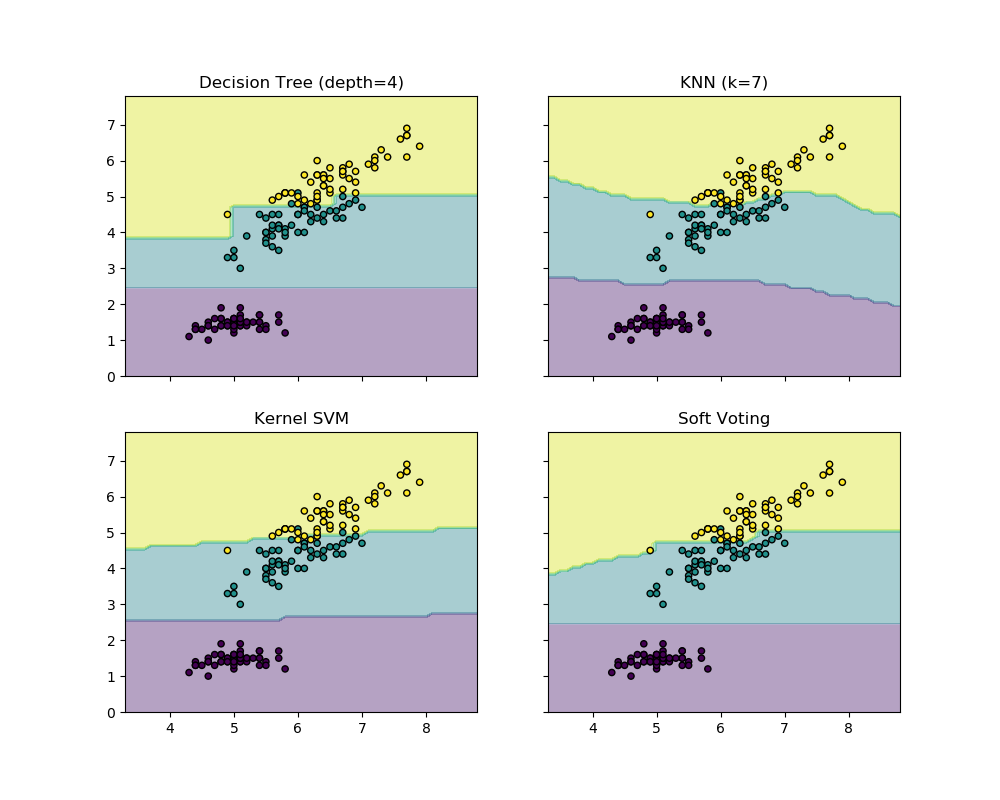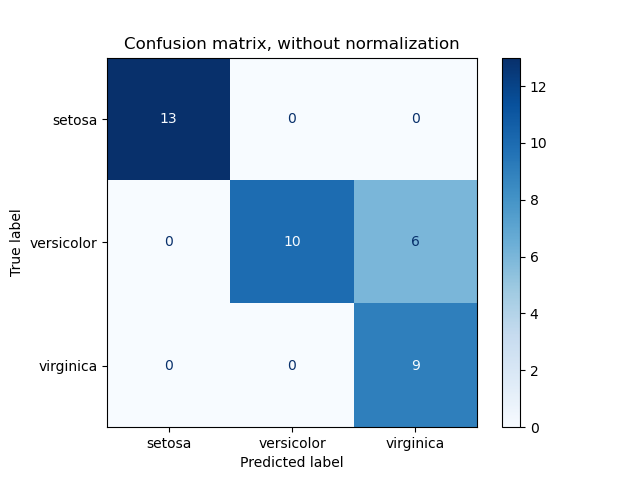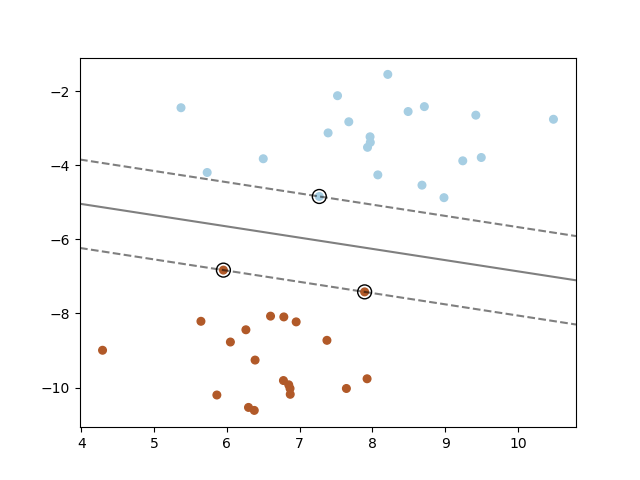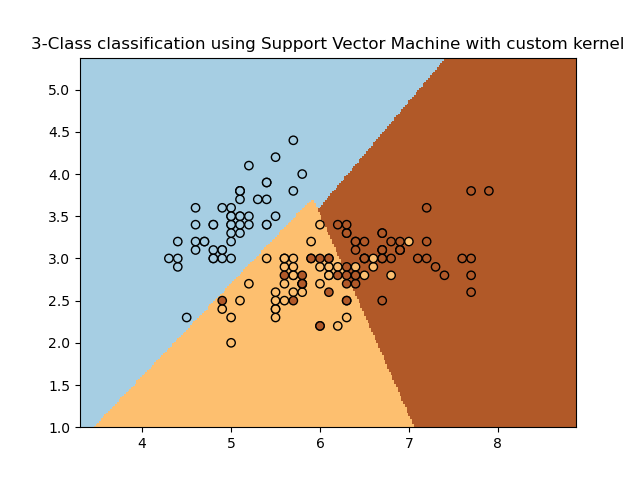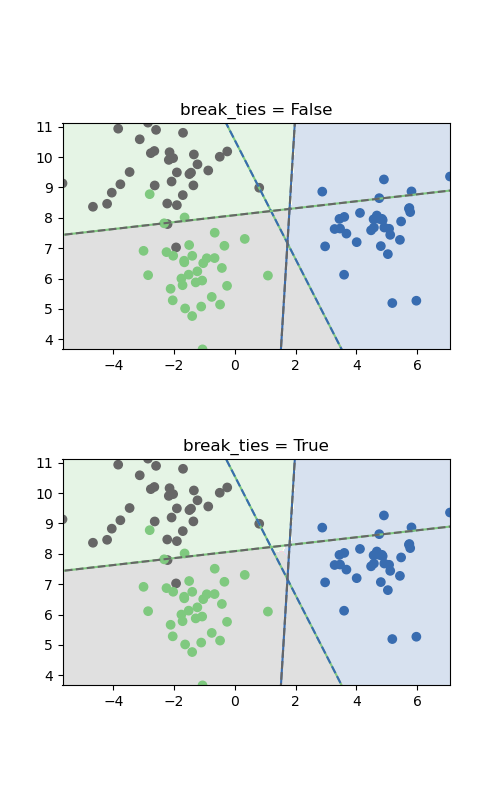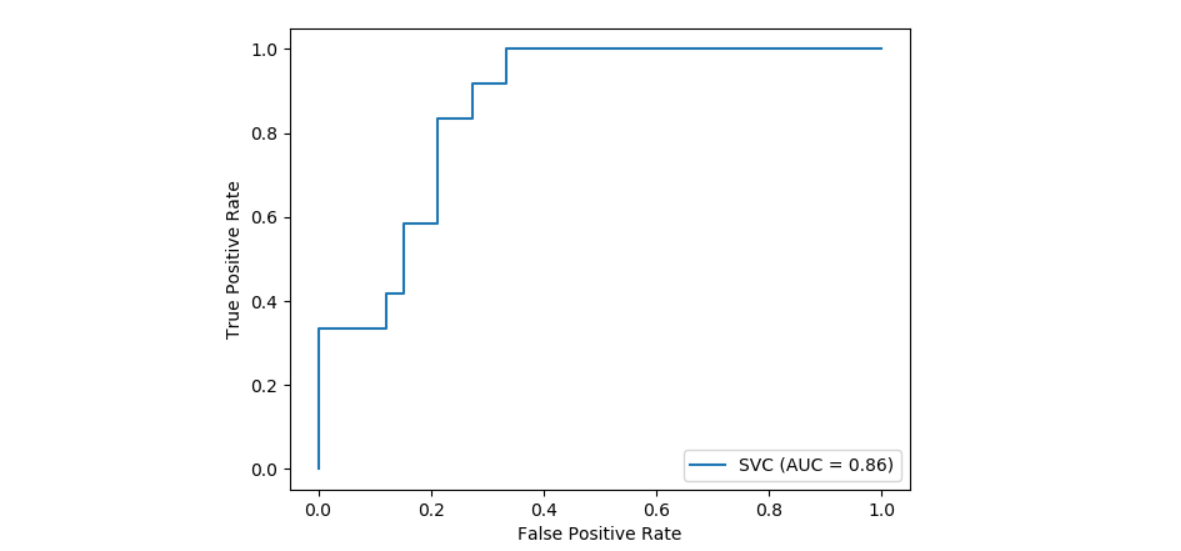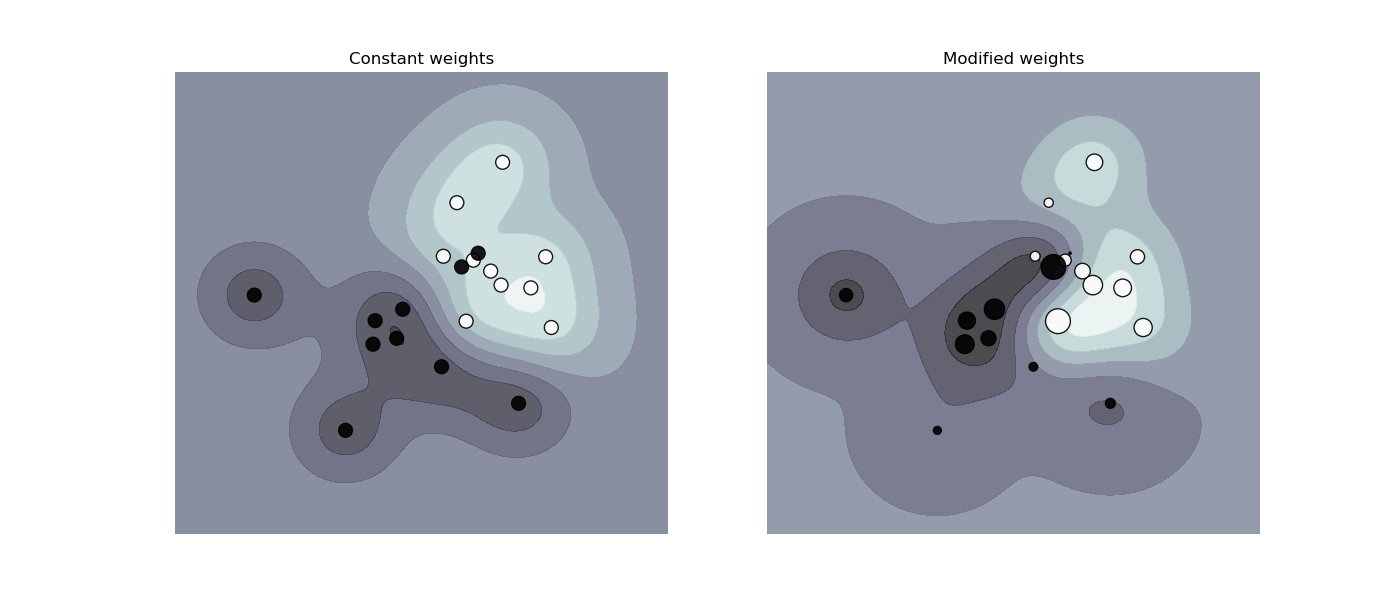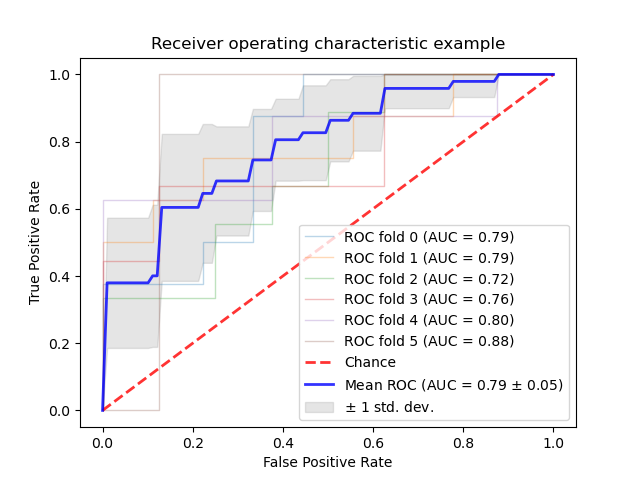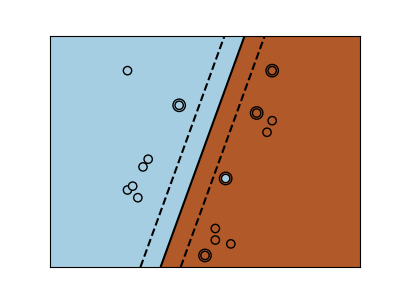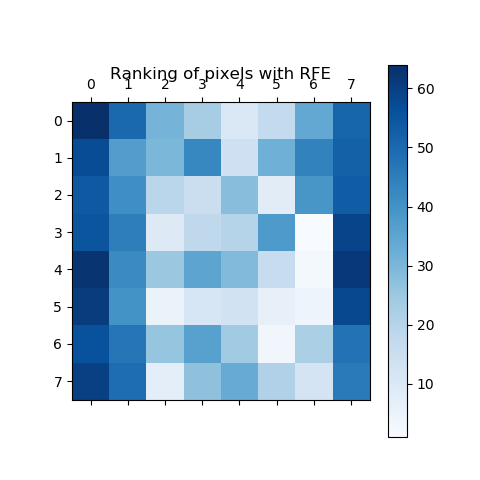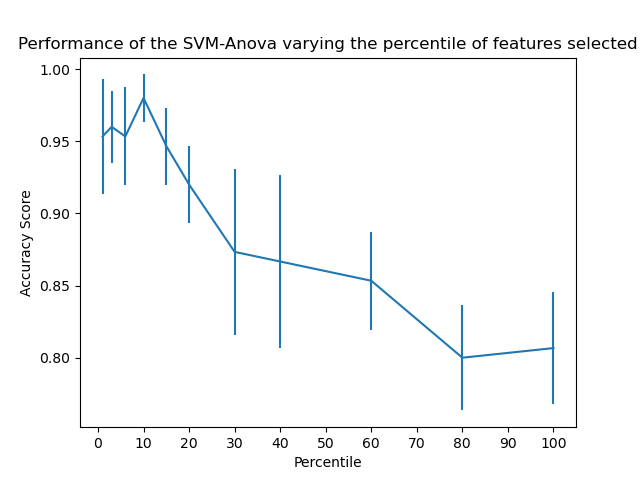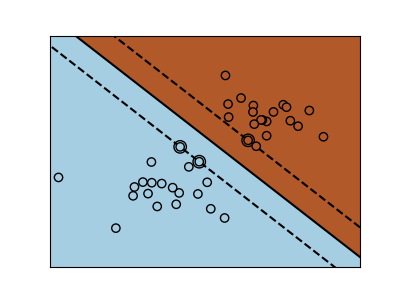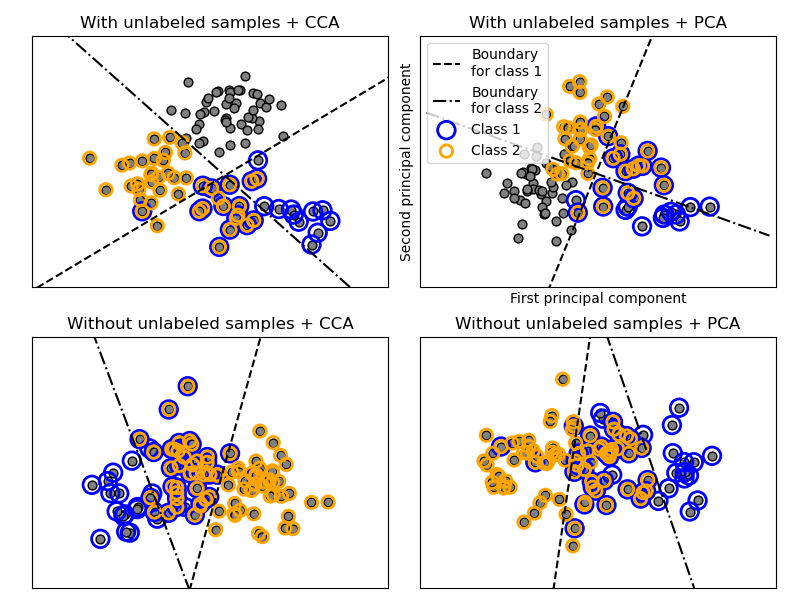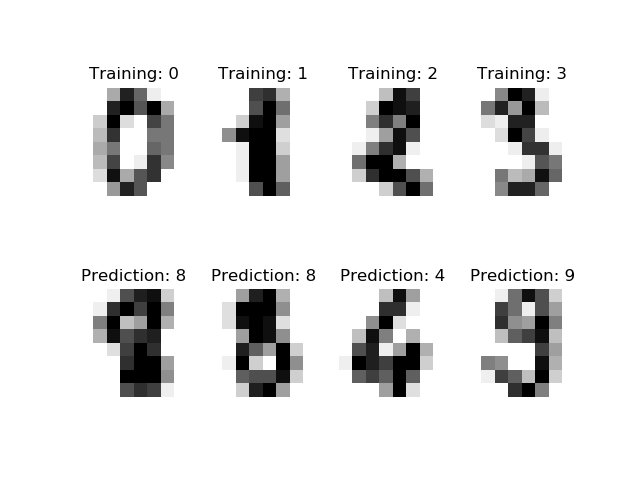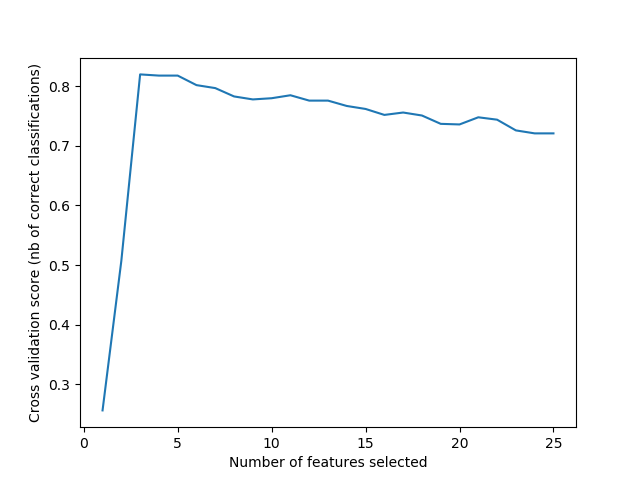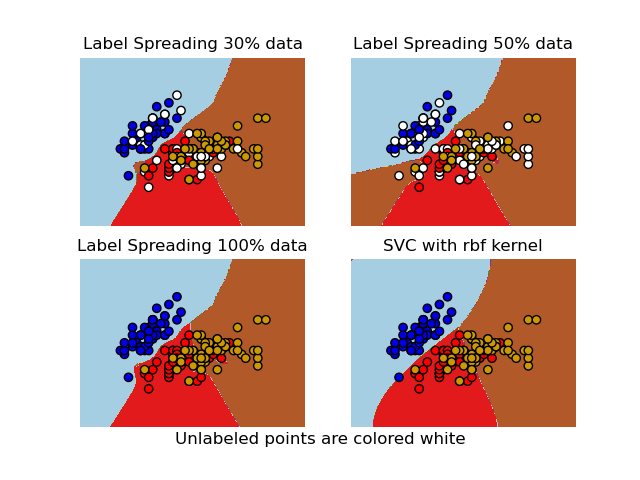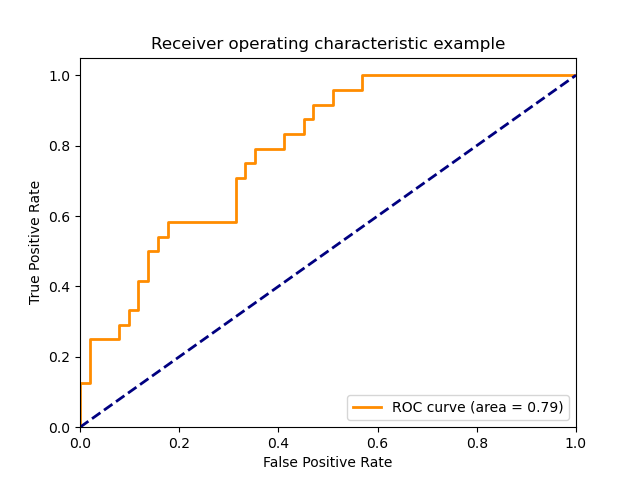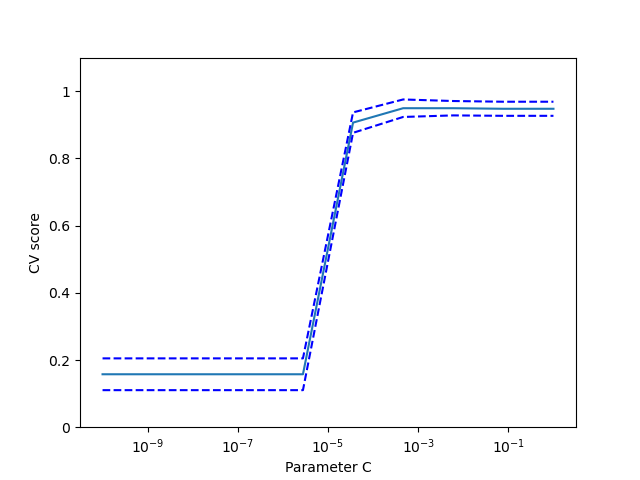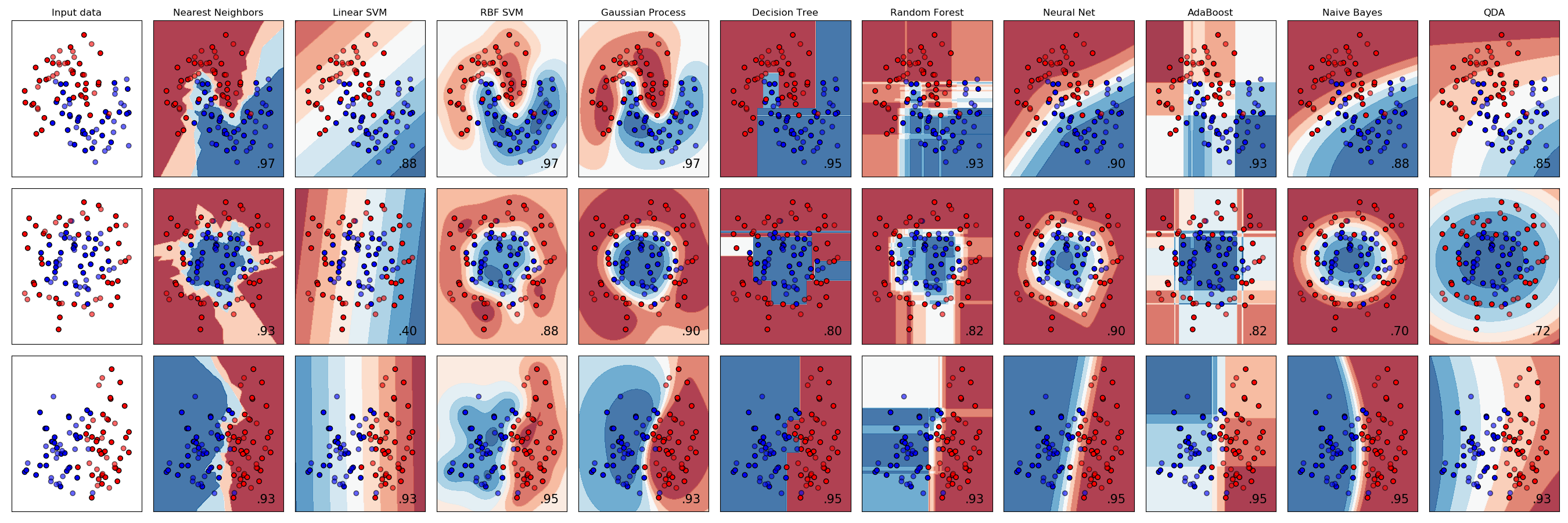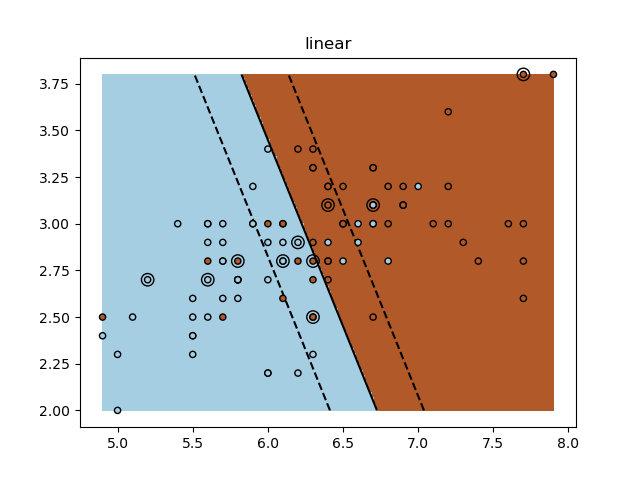sklearn.svm.SVC¶

class sklearn.svm.SVC(*, C=1.0, kernel='rbf', degree=3, gamma='scale', coef0=0.0, shrinking=True, probability=False, tol=0.001, cache_size=200, class_weight=None, verbose=False, max_iter=-1, decision_function_shape='ovr', break_ties=False, random_state=None)

C支持向量分类。

C 浮点数，默认= 1.0

kernel {‘linear’, ‘poly’, ‘rbf’, ‘sigmoid’, ‘precomputed’}, 默认=’rbf’

degree 整数型，默认=3

gamma 浮点数或者{‘scale’, ‘auto’} , 默认=’scale’

coef0 浮点数，默认=0.0

shrinking 布尔值，默认=True

probability 布尔值，默认=False

tol 浮点数，默认=1e-3

cache_size 浮点数，默认=200

class_weight {dict, ‘balanced’}, 默认=None

verbose 布尔值，默认=False

max_iter 整数型，默认=-1

decision_function_shape {‘ovo’, ‘ovr’}, 默认=’ovr’

0.17版中的新功能：推荐使用Decision_function_shape ='ovr'。

break_ties bool, default=False

random_state 整数型或RandomState的实例，默认=None

support_ 形如(n_SV,)的数组

support_vectors_ 形如(n_SV, n_features)的数组

n_support_ 形如(n_class)的数组，dtype=int32

dual_coef_ 形如(n_class-1, n_SV)的数组

coef_ 形如(n_class * (n_class-1) / 2, n_features)的数组

intercept_ 形如(n_class * (n_class-1) / 2,)的数组

fit_status_ 整数型

classes_ 形如(n_classes,)的数组

probA_ 形如(n_class * (n_class-1) / 2,)的数组
probB_ 形如(n_class * (n_class-1) / 2,)的数组

class_weight_ 形如(n_class,)的数组

shape_fit_ 形如(n_dimensions_of_X,)的整数型元祖

SVR

LinearSVC

>>> import numpy as np
>>> from sklearn.pipeline import make_pipeline
>>> from sklearn.preprocessing import StandardScaler
>>> X = np.array([[-1-1], [-2-1], , ])
>>> y = np.array()
>>> from sklearn.svm import SVC
>>> clf = make_pipeline(StandardScaler(), SVC(gamma='auto'))
>>> clf.fit(X, y)
Pipeline(steps=[('standardscaler', StandardScaler()),
('svc', SVC(gamma='auto'))])
>>> print(clf.predict([[-0.8-1]]))


decision_function(X) 计算X中样本的决策函数。
fit(X, y[, sample_weight]) 根据给定的训练数据拟合支持向量机模型。
get_params([deep]) 获取这个估计器的参数。
predict(X) 在X中对样本进行分类
score(X, y[, sample_weight]) 返回给定测试数据和标签的平均精度。
set_params(**params) 设置这个估计器的参数。
__init__(*, C=1.0, kernel='rbf', degree=3, gamma='scale', coef0=0.0, shrinking=True, probability=False, tol=0.001, cache_size=200, class_weight=None, verbose=False, max_iter=-1, decision_function_shape='ovr', break_ties=False, random_state=None)

X 形如(n_samples, n_features)的数组

X 形如 (n_samples, n_classes * (n_classes-1) / 2)的数组

fit(X, y, sample_weight=None)

X 形如(n_samples, n_features) 或者 (n_samples, n_samples)的数组或者稀疏矩阵

y 形如(n_samples,)的数组

sample_weight 形如(n_samples,)的数组，默认=None

self object

get_params(deep=True)

deep bool, default = True 如果为真，则将返回此估计量和作为估计量的所包含子对象的参数

params mapping of string to any 参数名被映射至他们的值
predict(X)

X 形如(n_samples, n_features) 或者 (n_samples_test, n_samples_train)的数组或者稀疏矩阵

y_pred 形如(n_sample, )的数组
X中样本的类别标签。
property predict_log_proba

X 形如(n_samples, n_features) 或者 (n_samples_test, n_samples_train)的数组或者稀疏矩阵

T 形如(n_sample, n_classes)的数组

property predict_proba

X 形如(n_samples, n_features)的数组

T 形如(n_sample, n_classes)的数组

score(X, y, sample_weight=None)

X 形如 (n_samples, nfeatures)的数组

y 形如 (n_sample, ) 或者 (n_samples, n_outputs)的数组
X中结果为真的标签
sample_weight 形如(n_samples,)的数组，默认=None

score 浮点型
self.predict(X) wrt. y.的平均精度
set_params(self, **params)

**f_params dict

self object

sklearn.svm.SVC使用示例¶